﻿ 胶接接头胶层切应力测试新方法 Experimental Study on Shear Stress of Adhesive Joint Layer

International Journal of Mechanics Research
Vol.07 No.01(2018), Article ID:24111,7 pages
10.12677/IJM.2018.71003

Experimental Study on Shear Stress of Adhesive Joint Layer

Yiwei Hua, Xuechen Li, Xuanchi Wu, Zhiyi Lin, Ming Xu

Civil Engineering College, Southeast University, Nanjing Jiangsu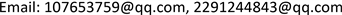Received: Feb. 28th, 2018; accepted: Mar. 14th, 2018; published: Mar. 21st, 2018ABSTRACT

Adhesive joint theoretical analysis shows the uneven distribution of layer shear stress. Many theories are formed to describe this distribution. But a test method to verify its theoretical result is absent. This paper presents a new layer shear stress test method. Through testing the normal stress of lap joint specimen, equation of normal stress distribution can be fitted. And then, the distribution of shear stress in internal layer can be deduced. The test results show that the adhesive shear stress distribution is consistent with the theoretical values.

Keywords:Adhesive Joint, Double Lap Joint, Adhesive Shear StressCopyright © 2018 by authors and Hans Publishers Inc.1. 引言

2. 测试方法

2.1. 测试原理

${\sigma }_{1x}=A\mathrm{cosh}\left(\lambda x\right)+B\mathrm{sinh}\left(\lambda x\right)+C$ (1)

$\tau =-\frac{\text{d}{\sigma }_{1x}}{\text{d}x}{t}_{1}=-{t}_{1}\lambda \left[A\mathrm{sinh}\left(\lambda x\right)+B\mathrm{cosh}\left(\lambda x\right)\right]$ (2)

${\lambda }^{2}=\frac{G}{t}\left(\frac{1}{{E}_{1}{t}_{1}}+\frac{1}{{E}_{2}{t}_{2}}\right)$

$A=\frac{F\left({E}_{2}{t}_{2}-{E}_{1}{t}_{1}\right)}{2{t}_{1}b\left({E}_{2}{t}_{2}+{E}_{1}{t}_{1}\right)\mathrm{cosh}\left(\lambda c\right)}$

$B=-\frac{F}{2{t}_{1}b\mathrm{sinh}\left(\lambda c\right)}$

$C=\frac{F{E}_{2}}{b\left({E}_{1}{t}_{1}+{E}_{2}{t}_{2}\right)}$

$\frac{\text{d}\sigma \left(x\right)}{\text{d}x}{t}_{1}+\tau \left(x\right)=0$ (3)

t1——盖板的厚度；

τ(x)——胶层中的切应力分布函数。

2.2. 测试方案

2.3. 测试过程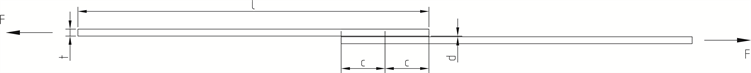Figure 1. Shear-Lag model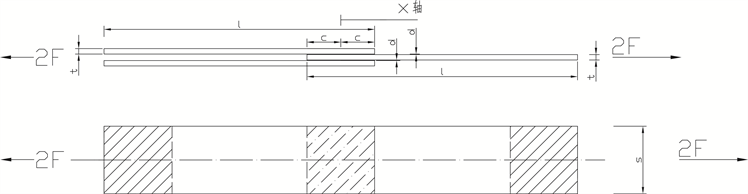Figure 2. Two-layer shear-Lag model

3. 测试结果

3.1. 盖板正应力测试结果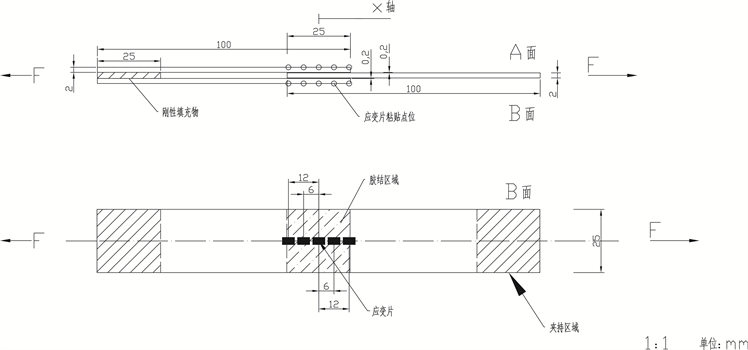Figure 3. Position of the strain gage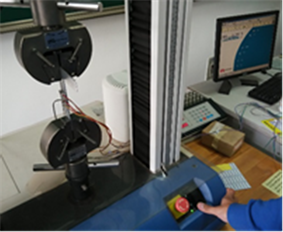Figure 4. Tension test of the samplesTable 3. Normal stress of the test position and its theoretical result

3.2. 胶层切应力测试结果

$\tau \left(x\right)=-{t}_{1}\lambda \left[A\mathrm{sinh}\lambda x+B\mathrm{cosh}\left(\lambda x\right)\right]$ (4)

${\alpha }_{l}=\frac{{\tau }_{\mathrm{max},l}}{{\tau }_{ave}},\text{\hspace{0.17em}}\text{\hspace{0.17em}}{\alpha }_{r}=\frac{{\tau }_{\mathrm{max},r}}{{\tau }_{ave}}$ (5)Table 4. The fitting result of normal stress distribution coefficientsTable 5. Test of the shear stress distribution and its theoretical resultFigure 5. Normal stress of the test position and its theoretical result

4. 结论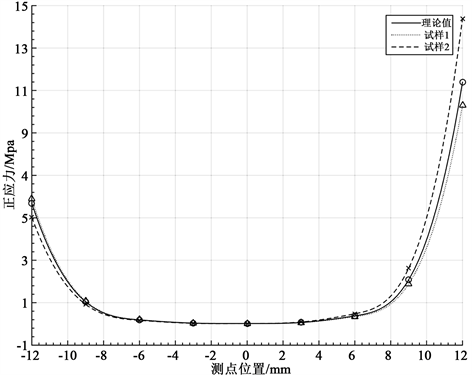Figure 6. Shear stress of the test position and its theoretical result

Experimental Study on Shear Stress of Adhesive Joint Layer[J]. 力学研究, 2018, 07(01): 19-25. https://doi.org/10.12677/IJM.2018.71003

1. 1. Volkersen, O. (1938) The Rivet Load Distribution in Lap-Joints with Members of Constant Thickness Subjected to Tension. Luftfahrtforschung, 15, 41-47.

2. 2. Goland, M. and Reissner, E. (1944) The Stresses in Cemented Joints. Journal of Apply Mechanic, 11, A17-A27.

3. 3. Ojalvo, I.U. and Eidinoff, H.L. (1978) Bond Thickness upon Stresses in Single-Lap Adhesive Joints. AIAA Journal, 16, 204-211.

4. 4. Adams, R.D. and Mallick, V. (1992) A Method for the Stress Analysis of Lap Joints. International Journal of Adhesion & Adhesives, 38, 199-217. https://doi.org/10.1080/00218469208030455

5. 5. Moutrille, M.P., Derrien, K., Baptiste, D., Balandraud, X. and Grédiac, M. (2009) Through-Thickness Strain Field Measurement in a Composite/Aluminum Adhesive Joint. Composites Part A, 40, 985-996. https://doi.org/10.1016/j.compositesa.2008.04.018